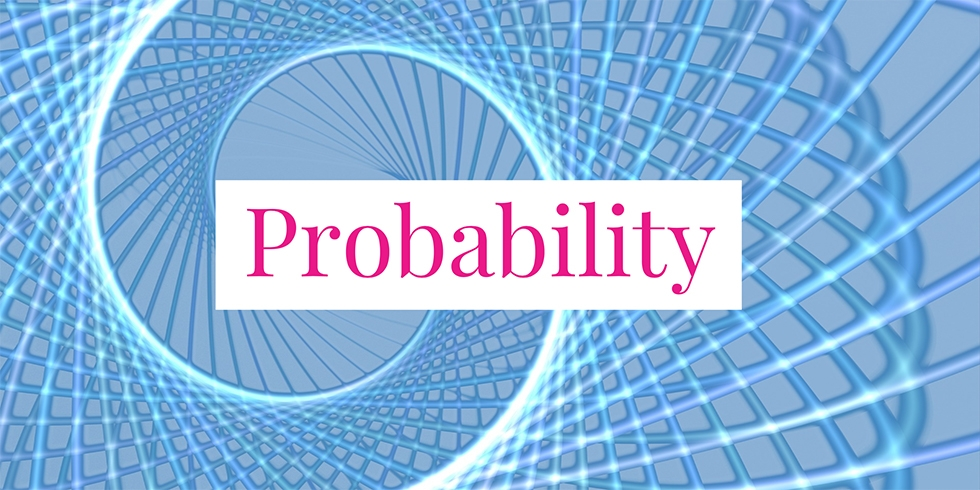# Independent and Dependent Events of Three Coins Tossing## Problem 733

Suppose that three fair coins are tossed. Let $H_1$ be the event that the first coin lands heads and let $H_2$ be the event that the second coin lands heads. Also, let $E$ be the event that exactly two coins lands heads in a row.

For each pair of these events, determine whether they are independent or not.Add to solve later

### Definition of Independence

Recall that events $E$ and $F$ are said to be independent if
$P(E \cap F) = P(E) P(F).$ Otherwise, they are dependent.

## Solution.

First of all, we have $P(H_1)= P(H_2)= 1/2$. To calculate the probability $P(E)$, note that we have $E = \{\text{hht}, \text{thh}\}$.
Here $\text{hht}$ means that the first and the second coins land heads and the third lands tails. Similarly for $\text{thh}$.

Thus,
$P(E)= \frac{2}{8} = \frac{1}{4}.$

Now we consider intersections of events.
First, since $H_1 \cap H_2 = \{\text{hhh}, \text{hht}\}$, we see that
$P(H_1 \cap H_2) = \frac{2}{8} = \frac{1}{4} = \frac{1}{2} \cdot \frac{1}{2} = P(H_1)\cdot P(H_2).$ Therefore, the events $H_1$ and $H_2$ are independent.

Next, as $H_1 \cap E = \{\text{hht}\}$, we have
$P(H_1 \cap E) = \frac{1}{8} = \frac{1}{2} \cdot \frac{1}{4} = P(H_1) \cdot P(E).$ Hence, the events $H_1$ and $E$ are independent.

Finally, since $H_2 \cap E = \{\text{hht}, \text{thh}\}$, we have
$P(H_2 \cap E) = \frac{2}{8} = \frac{1}{4}.$

On the other hand, we have
$P(H_2) \cdot P(E) = \frac{1}{2} \cdot \frac{1}{4} = \frac{1}{8}.$ It follows that $P(H_2 \cap E) \neq P(H_2) \cdot P(E)$.

Thus we conclude that the events $H_2$ and $E$ are dependent.Add to solve later

### More from my site

#### You may also like...

This site uses Akismet to reduce spam. Learn how your comment data is processed.

###### More in Probability##### Independent Events of Playing Cards

A card is chosen randomly from a deck of the standard 52 playing cards. Let $E$ be the event that...

Close